# Triangle Law of Vector Addition

## Statement of Triangle Law

If 2 vectors acting simultaneously on a body are represented both in magnitude and direction by 2 sides of a triangle taken in an order then the resultant(both magnitude and direction) of these vectors is given by 3rd side of that triangle taken in opposite order.

## Derivation of the law

Consider two vectors P and Q acting on a body and represented both in magnitude and direction by sides OA and AB respectively of a triangle OAB. Let θ be the angle between P and Q. Let R be the resultant of vectors P and Q. Then, according to triangle law of vector addition, side OB represents the resultant of P and Q.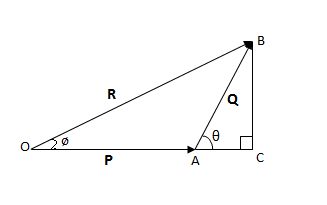So, we have

R = P + Q

Now, expand A to C and draw BC perpendicular to OC.

From triangle OCB,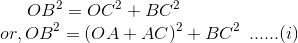In triangle ACB,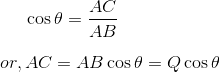Also,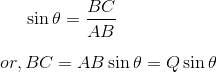Magnitude of resultant:

Substituting value of AC and BC in (i), we get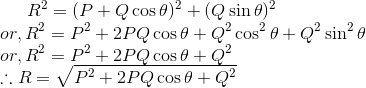which is the magnitude of resultant.

Direction of resultant: Let ø be the angle made by resultant R with P. Then,

From triangle OBC,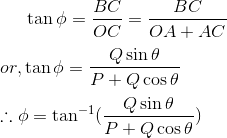which is the direction of resultant.